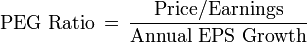# PEG Ratio

The PEG ratio (price/earnings to growth ratio) is a valuation metric for determining the relative trade-off between the price of a stock, the earnings generated per share (EPS), and the company’s expected growth.

In general, the P/E ratio is higher for a company with a higher growth rate. Thus using just the P/E ratio would make high-growth companies appear overvalued relative to others. It is assumed that by dividing the P/E ratio by the earnings growth rate, the resulting ratio is better for comparing companies with different growth rates.The P/E ratio used in the calculation may be projected or trailing, and the annual growth rate may be the expected growth rate for the next year or the next five years.I t is favoured by many over the price/earnings ratio because it also accounts for growth.

The PEG ratio is less appropriate for measuring companies without high growth. PEG is a widely employed indicator of a stock’s possible true value. A fairly valued company will have its PEG equal to 1, indicating that a stock is reasonably valued given the expected growth. Similar to PE ratios, a lower PEG means that the stock is undervalued more and may provide higher returns. The PEG Ratio can also be a negative number, for example, when earnings are expected to decline.

Example: Google’s PEG ratio is 0.95 (5 year expected)